Art by Peter Olofsson

There are two reasons I picked this puzzle for writing a tutorial: (1) I’m a fan of the Terminator series, and (2) after many years, I was successfully able to write a Depth-First Search algorithm (DFS) that solved an interesting problem. There are probably better solutions out there, but I’d like to share how I implemented mine.

## Problem Statement and the Goal – In Graph Terms

Given –

1. an undirected graph
2. a root node (source) (i.e., where the skynet agent starts)
3. multiple destination nodes (i.e., gateways)

In each game turn, the program should cut off an edge between two nodes where at least one of these nodes is a destination node so that at the end of the game all destination nodes are unreachable.

The following actions take place at every turn:

1. The player cuts a link.
2. A new source node is selected (the skynet agent moves to an adjacent node).

I’m going to explain the problem and the goal with the constraint in an image below. Consider the following graph with 4 nodes where

• The red node is the source
• The green nodes are gateways
• The links that can be cut are blue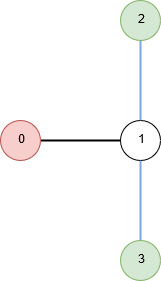## Initial Thoughts on the Solution

For the above graph, the player can cut either of the links in the first turn. The agent then moves to node 1. In the second turn, the player cuts the other link.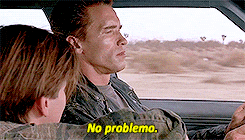Easy, right?

Notice that you never want the agent to reach node 1 while node 1 is still connected with nodes 2 and 3. In that case, no matter what you cut, you’ll lose the game during the agent’s turn.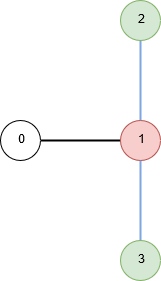So the agent will always try to reach a node from which more than one gateway is reachable. You can think of this as the winning move for the agent (which makes you lose the game).

Also, the agent will try to force you to make specific moves. For example, in the 7 node graph below where 2, 3 and 6 are gateways, starting from node 0 the agent will move to 1 to force the player to cut 1-3 immediately.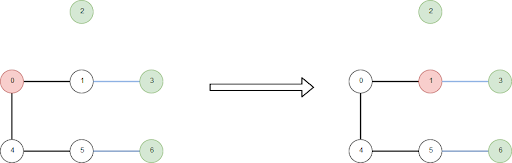Combining the above two strategies, the agent can reach its desired gateway by the following algorithm each turn:

1. Try to reach a node from which more than 1 gateway is connected.
2. If the node in (1) isn’t available, try to reach a node connected to at least 1 gateway.
3. If the node in (2) isn’t available, try to reach a node from which a gateway is closest.

## Thinking as the Agent

The following graph is inspired from test case 5. The number beside each node indicates the distance from that node to the closest gateway.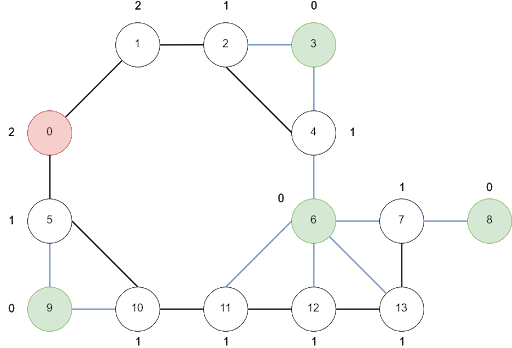Notice nodes 4 and 7 – they are connected to 2 gateways. They are highly desirable for the agent to reach since they are connected to 2 gateways. The shortest distance from 0 to 4 is 3, and from 0 to 7 is 5. It makes it tempting to cut either 4-3 or 4-6 link in the first turn. But that would be a deadly mistake. Why?

Suppose we cut either 4-3 or 4-6 in the first turn. The agent will move as follows:

 Agent Player 1 Moves to 5. Forced to cut 5-9. 2 Moves to 10. Forced to cut 10-9. 3 Moves to 11. Forced to cut 11-6. 4 Moves to 12. Forced to cut 12-6. 5 Moves to 13. Forced to cut 13-6. 6 Moves to 7. Any move will make the agent win in the next turn.

Any move will make the agent win in the next turn.

## Forging the Solution

Now that we understand how the agent behaves, how can we counter the attack? As the agent moves to the node closest to a gateway, we need to compute the distance to the closest gateway for every node. It can be achieved with a BFS algorithm. Let’s define the following function:

```GetDistanceToClosestGateway(graph, gateways) -> dist_closest_gw

for each node n in graph
dist_closest_gw[n] = INFINITY

for each gateway gw in gateways
dist_closest_gw[gw] = 0

initialize a queue, each element of queue is a node number
queue.push(gw)

while(queue isn’t empty)
source = queue.front()
for each node i in graph
if i is reachable from source and
i is never visited
idist = dist_closest_gw[source] + 1
if idist < dist_closest_gw[i]
dist_closest_gw[i] = idist
queue.push(i)
return dist_closest_gw```

It takes the graph and the list of gateways as parameters and returns an array containing the distance to the closest gateway for each node.

Next we define a function that will return an array that indicates the number of gateways connected to each node:

`GetConnectedGatewayCount(graph, gateways) -> connected_gw`

After the execution of the function above, connected_gw[i] will indicate the number of gateways connected to node i. It’s easy enough to implement, so I’m skipping the explanation of the implementation.

## Thinking as the Player

Notice the example above. The agent chose to visit node 7 instead of node 4 even though node 4 is nearer. This is because the player is handicapped by the agent’s moves if the agent moves to node 5 – after each of the agent’s turns, the player is forced to cut a specific link. Being more intelligent, we can’t let that happen.

Think of it this way – how quickly (i.e., minimum number of turns) will the agent start forcing the player to cut a specific link? If the agent moves to node 1, then the agent can’t force anything; the agent needs to move to node 2 to start forcing the player. That’s 2 moves. If the agent moves to node 5, then the player is already under pressure and is forced to cut specific links (i.e., 5-9). That’s 1 move. That’s the beginning of the winning moves for the agent.

Let’s write the following function to determine this shortest turn. It’s a BFS algorithm with a twist.

```GetShortestTurnCost(graph, source, connected_gw, gateways) -> cost

for each node n in graph
cost[n] = INFINITY
initialize a queue; each element of the queue is a node and its parent
queue.push(source, -1)
cost[source] = 0

while (queue is not empty)
cur_node, parent = q.front()

//no need to consider paths that contains gateway
if cur_node is a gateway, continue with the next element

for each node i in graph
if i is reachable from cur_node and i is never visited
if connected_gw[i] > 0 and parent >= 0
new_cost = cost[cur_node]
else
new_cost = cost[cur_node] + 1

if new_cost < cost[i]
cost[i] = new_cost
queue.push(i, cur_node)

return cost```

Now the final piece. I used a DFS to simulate the agent’s moves following the algorithm stated in the initial thoughts section. As the agent, the DFS will try to find a node i for which connected_gw[i] > 1. This is the winning condition of the agent. To force the player to cut specific links, the agent needs to move among nodes j to reach i for which connected_gw[j] == 1. If the agent is forced to move to a node j such that connected_gw[j] == 0, the player will use that turn to reduce connected_gw[i] and the agent will not be successful.

## The DFS Algorithm

Take a look at the graph above. We know that the agent will select node 5 in the next turn, not 1. Another way to think about that is to note that the distance to the closest gateway from node 5 is 1, whereas from node 1 it’s 2. So when designing the solution in DFS, we can’t select an arbitrary adjacent vertex to jump to – we need to prioritize where to jump next from the current node. From the current node (where the agent is on the current turn) we need to put all connecting nodes in a priority queue. We need to select the next node from the priority queue of connecting nodes that we’ll have sorted beforehand.

The priority queue should sort the nodes as follows:

1. By number of connected gateways (decreasing)
2. By the distance to the closest gateway (increasing)

Finally, here is the DFS I used. It has the following parameters:

• source: The agent’s starting/current node
• cur_node: The node currently visited
• graph: The given graph
• connected_gw: connected_gw[i] represents the number of gateways connected to node i
• dist_closest_gw: dist_closest_gw[i] represents the distance from node i to the closest gateway
• vis: vis[i] is true if node i has been visited, false otherwise
• discard: true if a gateway is reachable or if a node is reachable from which more than 1 gateway is reachable
• shortest_cost: from agent’s current node to node i, shortest_cost[i] represents minimum number of edges for which no node in those edges is a gateway
```Dfs(source, cur_node, graph, connected_gw,

initialize the priority queue
vis[cur_node] = true

for each node i reachable from cur_node and not visited
if connected_gw[i] > 0
// Only traverse through nodes which is connected to a gateway
// Otherwise we’ve time to cut links
enqueue node i and store connected_gw[i] and dist_closest_gw[i]
vis[i] = true

if source and cur_node are same and
dist_closest_gw[i] == 0 // i is a gateway
discard = true // A gateway is reachable from source
return

while priority queue is not empty
node, connected_gw_count = front of priority queue
if connected_gw[node] > shortest_cost[node]
// A node from which number of reachable gateways is
// greater than cost to reach the node
return

Dfs(source, node, graph, connected_gw, dist_closest_gw, vis, discard, shortest_cost)```

## Final Thoughts

That’s all. Figuring out the DFS along with the utilization of a priority queue was the challenging part for me, and once I figured that out, I was pretty excited. I hope you’ll find this tutorial useful to start developing a solution of your own.